Скачать презентацию CHAPTER TWENTY-FOUR OPTIONS 1 TYPES OF OPTION

b41b51a82862370a7786495757ef9f19.ppt

• Количество слайдов: 49CHAPTER TWENTY-FOUR OPTIONS 1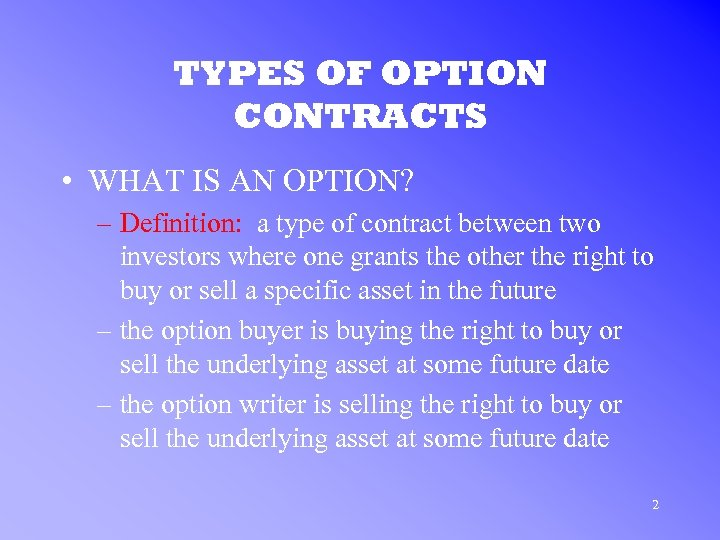TYPES OF OPTION CONTRACTS • WHAT IS AN OPTION? – Definition: a type of contract between two investors where one grants the other the right to buy or sell a specific asset in the future – the option buyer is buying the right to buy or sell the underlying asset at some future date – the option writer is selling the right to buy or sell the underlying asset at some future date 2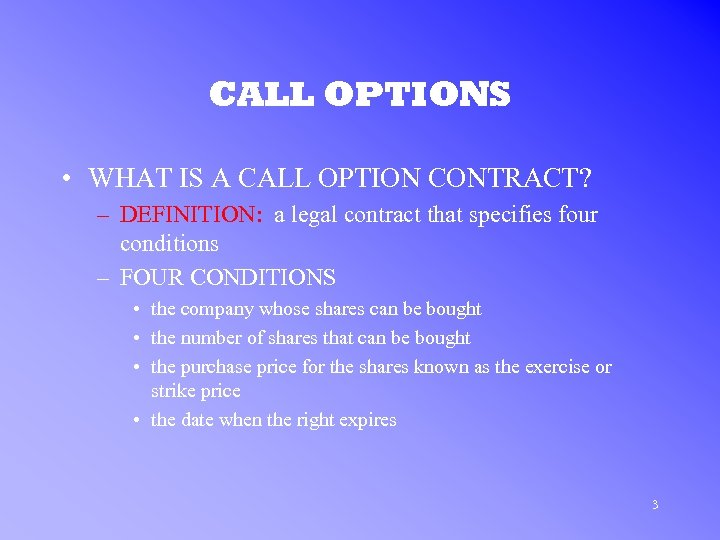CALL OPTIONS • WHAT IS A CALL OPTION CONTRACT? – DEFINITION: a legal contract that specifies four conditions – FOUR CONDITIONS • the company whose shares can be bought • the number of shares that can be bought • the purchase price for the shares known as the exercise or strike price • the date when the right expires 3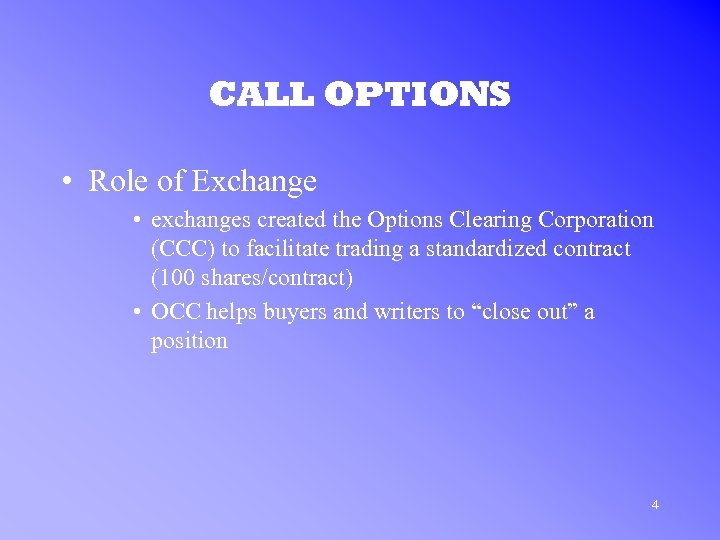CALL OPTIONS • Role of Exchange • exchanges created the Options Clearing Corporation (CCC) to facilitate trading a standardized contract (100 shares/contract) • OCC helps buyers and writers to “close out” a position 4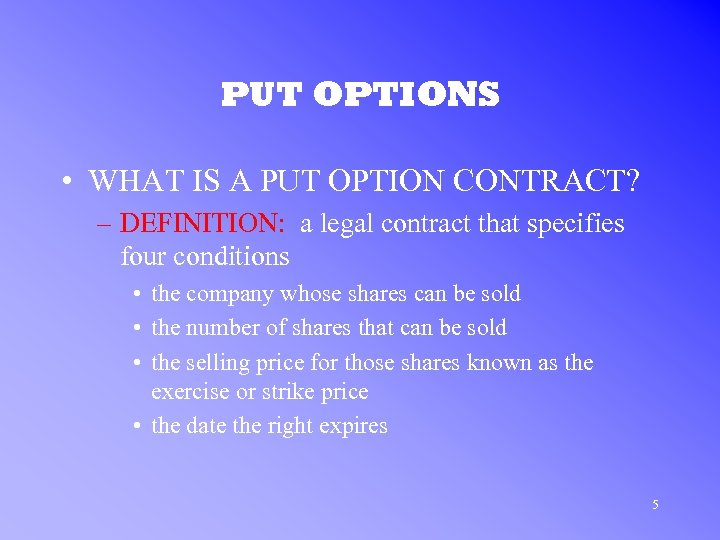PUT OPTIONS • WHAT IS A PUT OPTION CONTRACT? – DEFINITION: a legal contract that specifies four conditions • the company whose shares can be sold • the number of shares that can be sold • the selling price for those shares known as the exercise or strike price • the date the right expires 5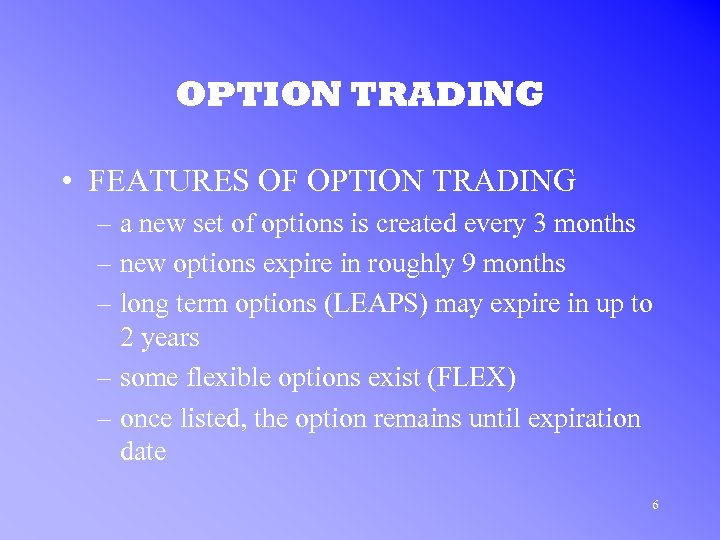OPTION TRADING • FEATURES OF OPTION TRADING – a new set of options is created every 3 months – new options expire in roughly 9 months – long term options (LEAPS) may expire in up to 2 years – some flexible options exist (FLEX) – once listed, the option remains until expiration date 6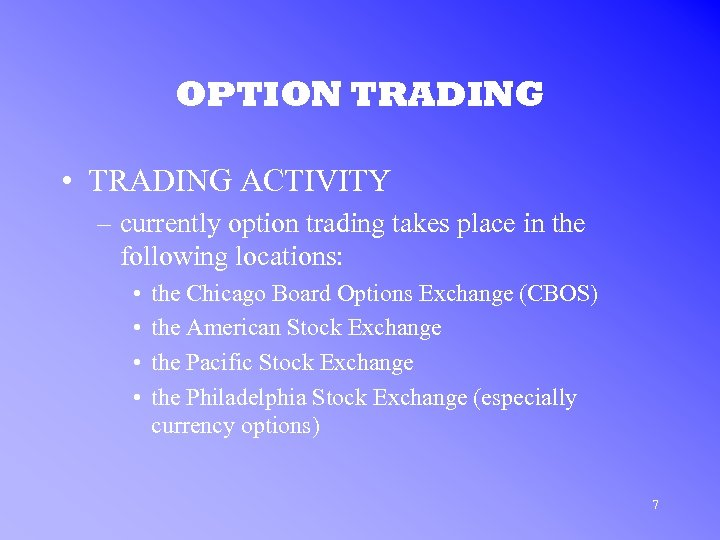OPTION TRADING • TRADING ACTIVITY – currently option trading takes place in the following locations: • • the Chicago Board Options Exchange (CBOS) the American Stock Exchange the Pacific Stock Exchange the Philadelphia Stock Exchange (especially currency options) 7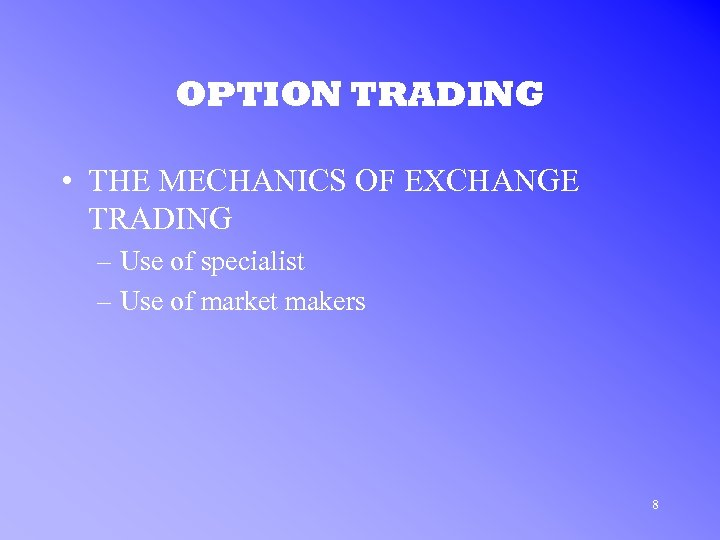OPTION TRADING • THE MECHANICS OF EXCHANGE TRADING – Use of specialist – Use of market makers 8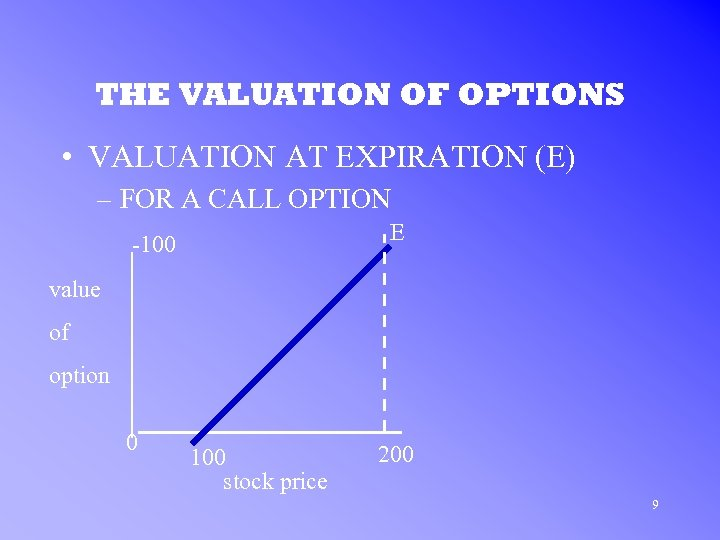THE VALUATION OF OPTIONS • VALUATION AT EXPIRATION (E) – FOR A CALL OPTION E -100 value of option 0 100 stock price 200 9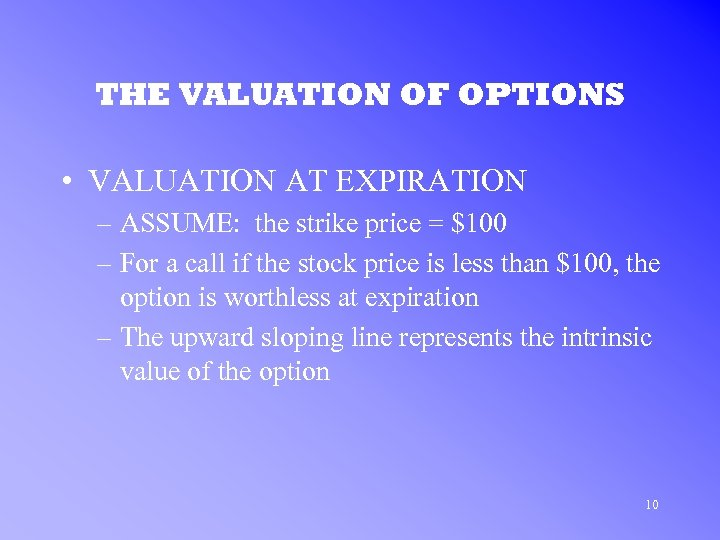THE VALUATION OF OPTIONS • VALUATION AT EXPIRATION – ASSUME: the strike price = \$100 – For a call if the stock price is less than \$100, the option is worthless at expiration – The upward sloping line represents the intrinsic value of the option 10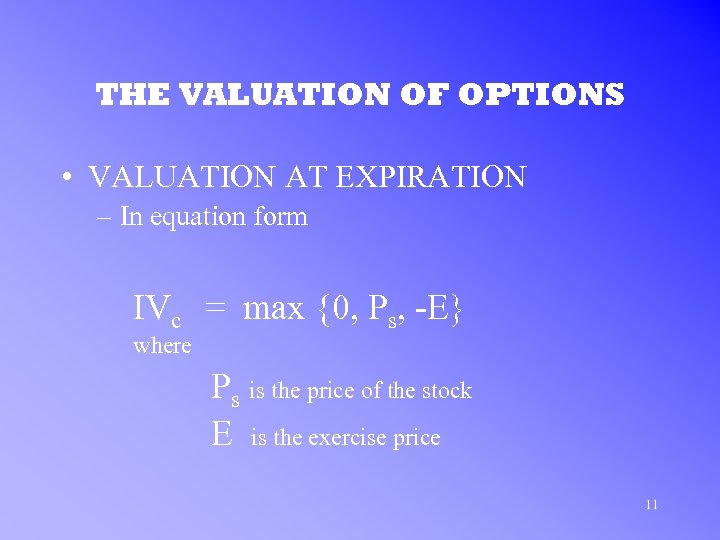THE VALUATION OF OPTIONS • VALUATION AT EXPIRATION – In equation form IVc = max {0, Ps, -E} where Ps is the price of the stock E is the exercise price 11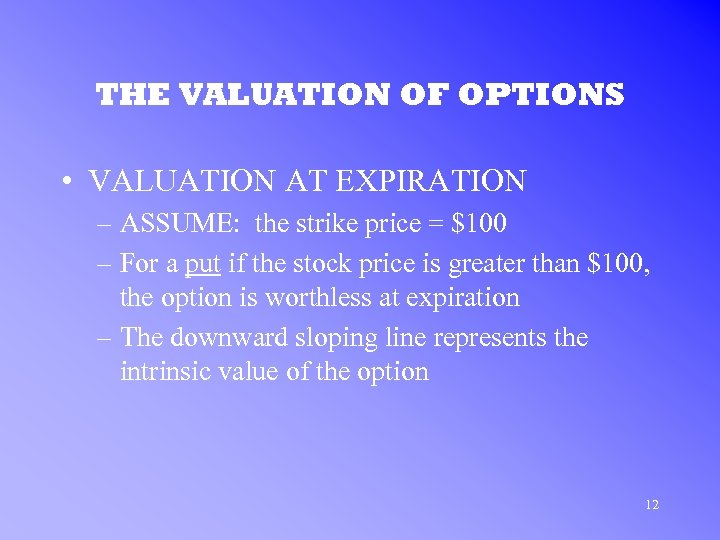THE VALUATION OF OPTIONS • VALUATION AT EXPIRATION – ASSUME: the strike price = \$100 – For a put if the stock price is greater than \$100, the option is worthless at expiration – The downward sloping line represents the intrinsic value of the option 12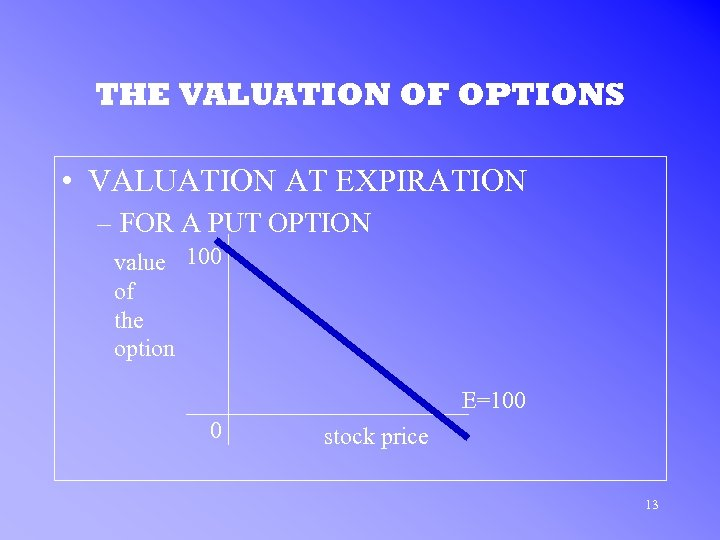THE VALUATION OF OPTIONS • VALUATION AT EXPIRATION – FOR A PUT OPTION value 100 of the option E=100 0 stock price 13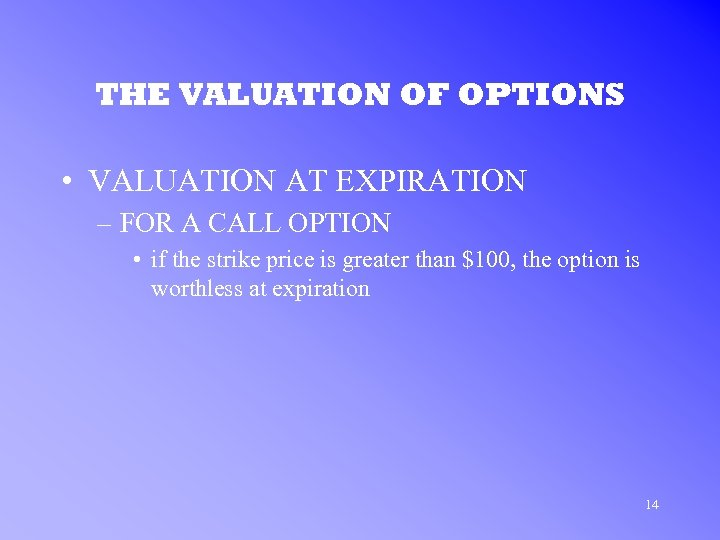THE VALUATION OF OPTIONS • VALUATION AT EXPIRATION – FOR A CALL OPTION • if the strike price is greater than \$100, the option is worthless at expiration 14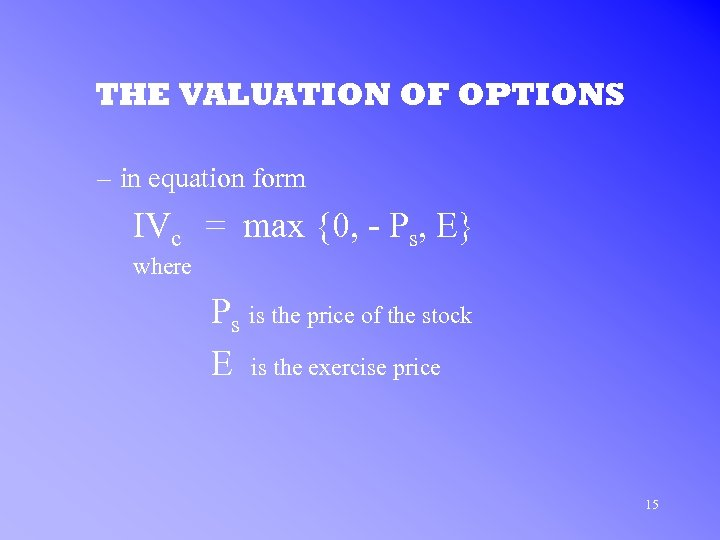THE VALUATION OF OPTIONS – in equation form IVc = max {0, - Ps, E} where Ps is the price of the stock E is the exercise price 15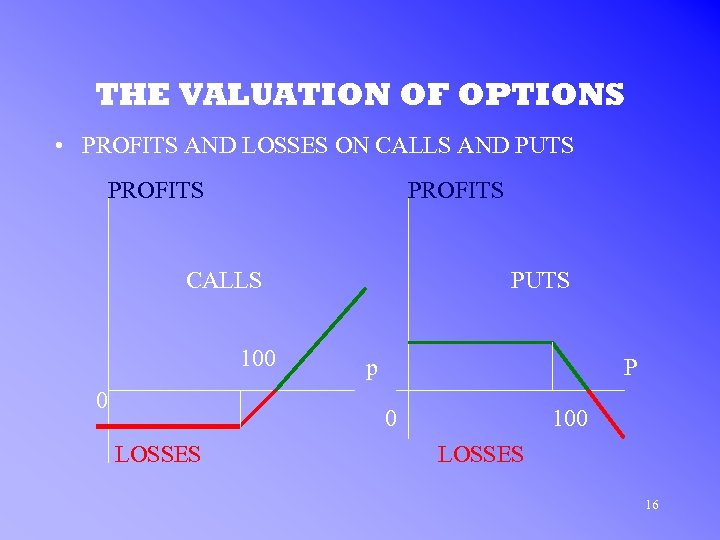THE VALUATION OF OPTIONS • PROFITS AND LOSSES ON CALLS AND PUTS PROFITS CALLS 100 0 PUTS p P 0 LOSSES 100 LOSSES 16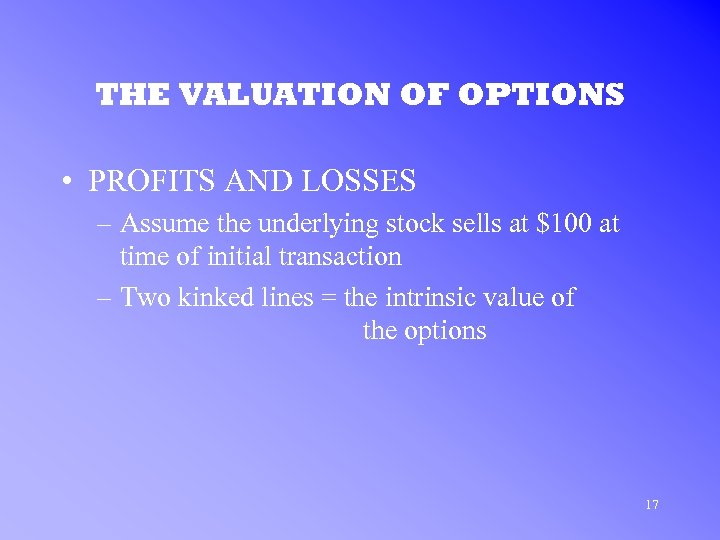THE VALUATION OF OPTIONS • PROFITS AND LOSSES – Assume the underlying stock sells at \$100 at time of initial transaction – Two kinked lines = the intrinsic value of the options 17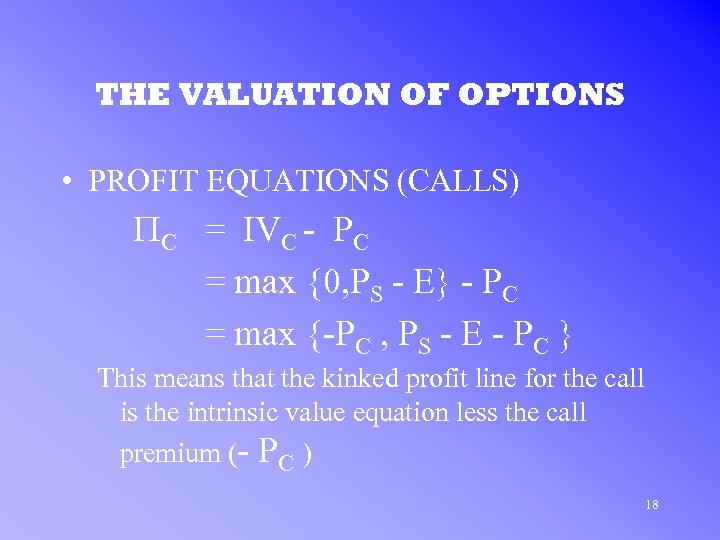THE VALUATION OF OPTIONS • PROFIT EQUATIONS (CALLS) PC = IVC - PC = max {0, PS - E} - PC = max {-PC , PS - E - PC } This means that the kinked profit line for the call is the intrinsic value equation less the call premium (- PC ) 18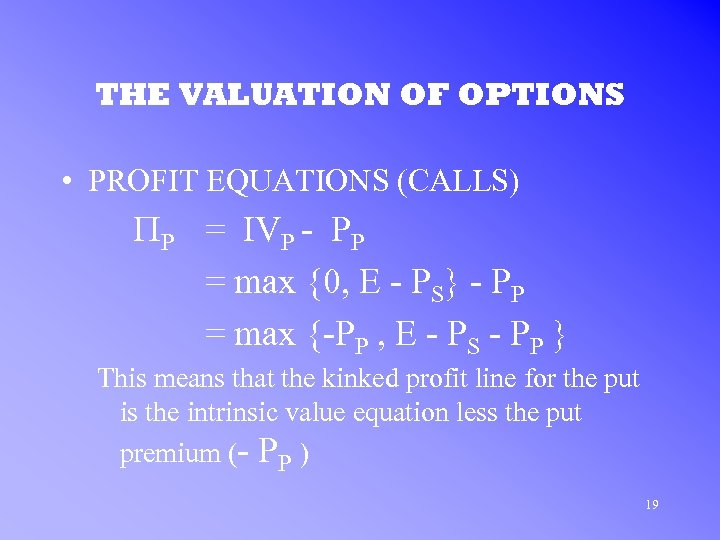THE VALUATION OF OPTIONS • PROFIT EQUATIONS (CALLS) PP = IVP - PP = max {0, E - PS} - PP = max {-PP , E - PS - PP } This means that the kinked profit line for the put is the intrinsic value equation less the put premium (- PP ) 19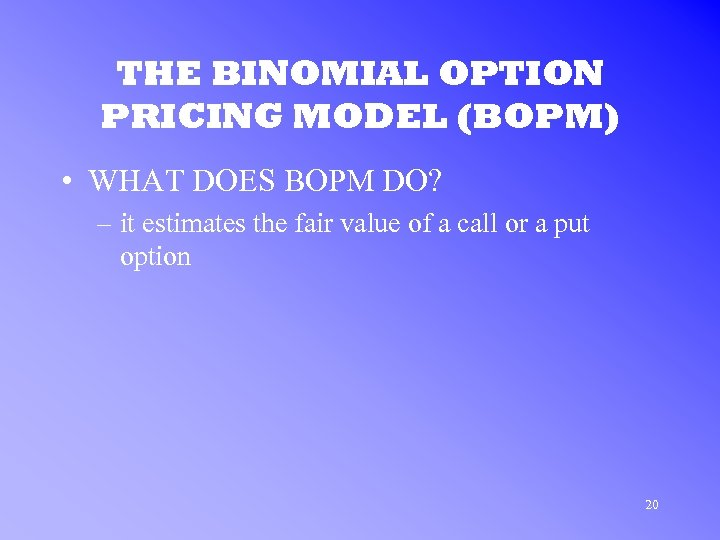THE BINOMIAL OPTION PRICING MODEL (BOPM) • WHAT DOES BOPM DO? – it estimates the fair value of a call or a put option 20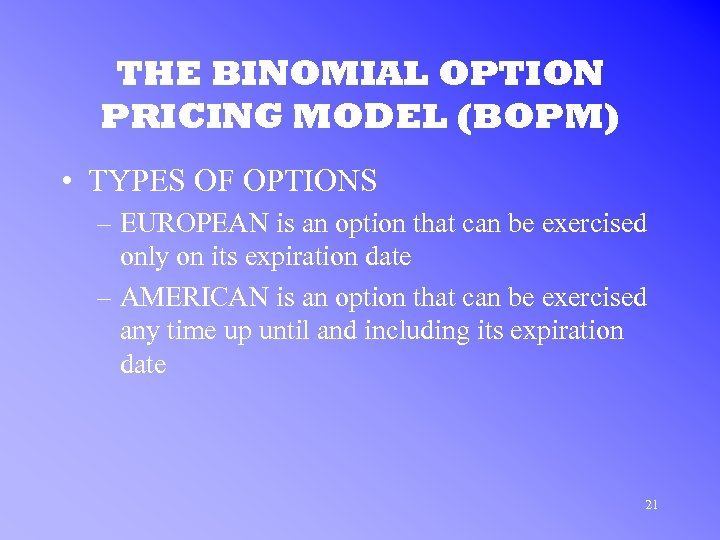THE BINOMIAL OPTION PRICING MODEL (BOPM) • TYPES OF OPTIONS – EUROPEAN is an option that can be exercised only on its expiration date – AMERICAN is an option that can be exercised any time up until and including its expiration date 21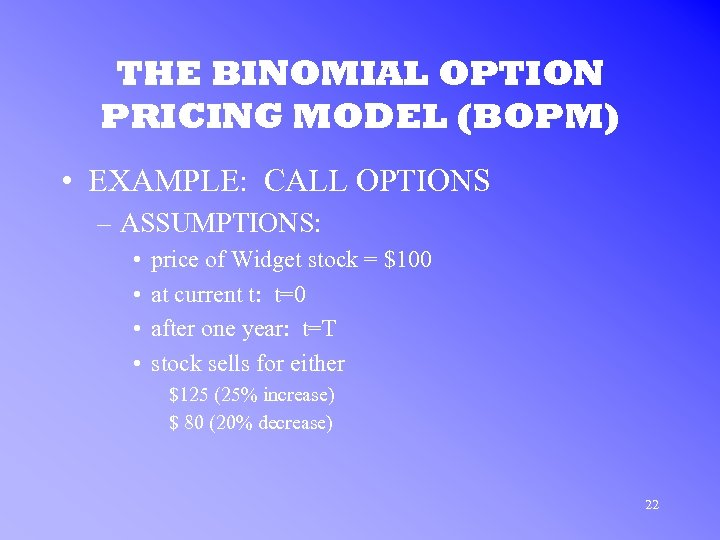THE BINOMIAL OPTION PRICING MODEL (BOPM) • EXAMPLE: CALL OPTIONS – ASSUMPTIONS: • • price of Widget stock = \$100 at current t: t=0 after one year: t=T stock sells for either \$125 (25% increase) \$ 80 (20% decrease) 22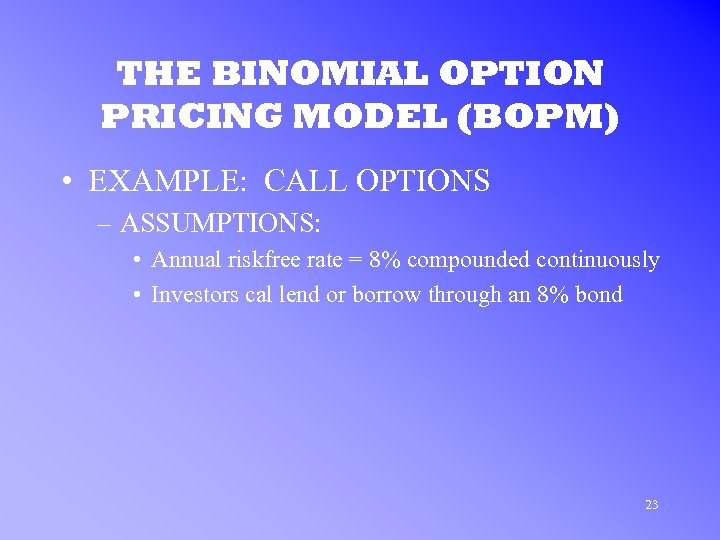THE BINOMIAL OPTION PRICING MODEL (BOPM) • EXAMPLE: CALL OPTIONS – ASSUMPTIONS: • Annual riskfree rate = 8% compounded continuously • Investors cal lend or borrow through an 8% bond 23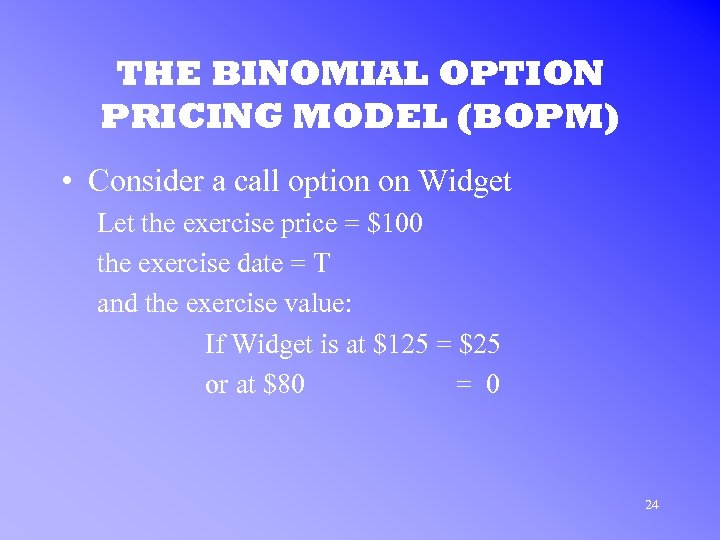THE BINOMIAL OPTION PRICING MODEL (BOPM) • Consider a call option on Widget Let the exercise price = \$100 the exercise date = T and the exercise value: If Widget is at \$125 = \$25 or at \$80 = 0 24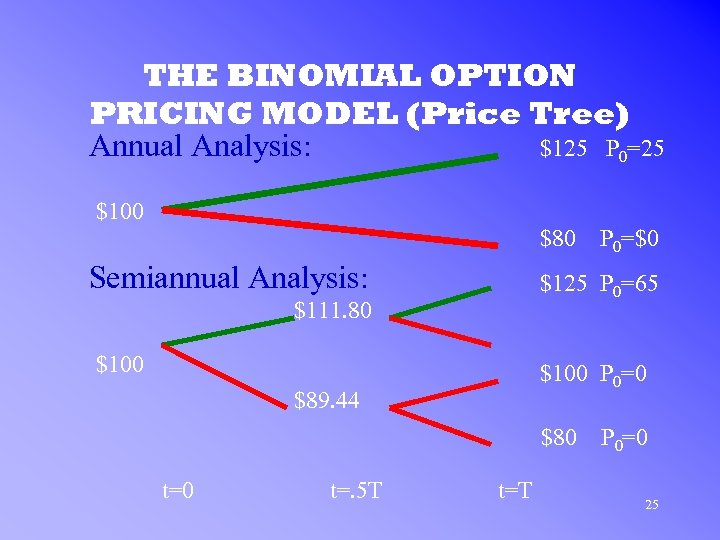THE BINOMIAL OPTION PRICING MODEL (Price Tree) Annual Analysis: \$125 P 0=25 \$100 \$80 Semiannual Analysis: \$125 P 0=65 \$111. 80 \$100 P 0=0 \$89. 44 \$80 t=. 5 T P 0=\$0 t=T P 0=0 25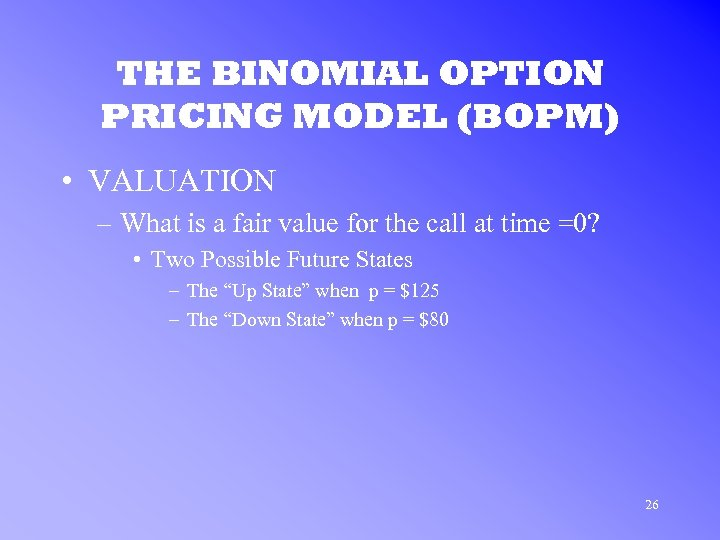THE BINOMIAL OPTION PRICING MODEL (BOPM) • VALUATION – What is a fair value for the call at time =0? • Two Possible Future States – The “Up State” when p = \$125 – The “Down State” when p = \$80 26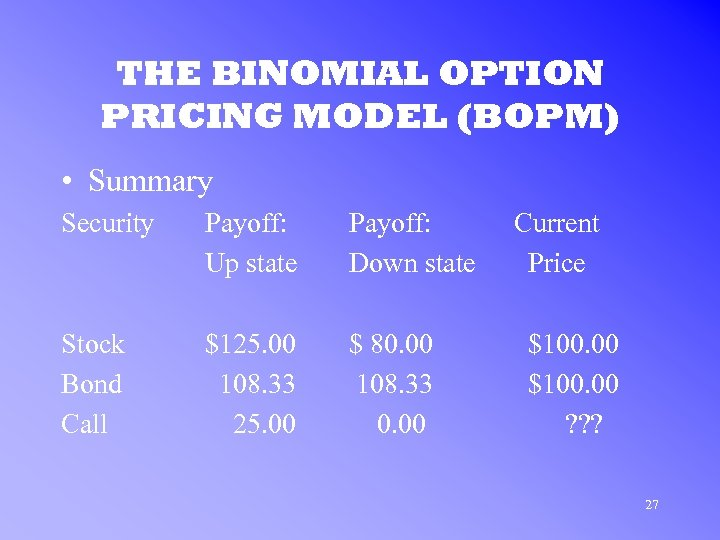THE BINOMIAL OPTION PRICING MODEL (BOPM) • Summary Security Payoff: Up state Payoff: Down state Stock Bond Call \$125. 00 108. 33 25. 00 \$ 80. 00 108. 33 0. 00 Current Price \$100. 00 ? ? ? 27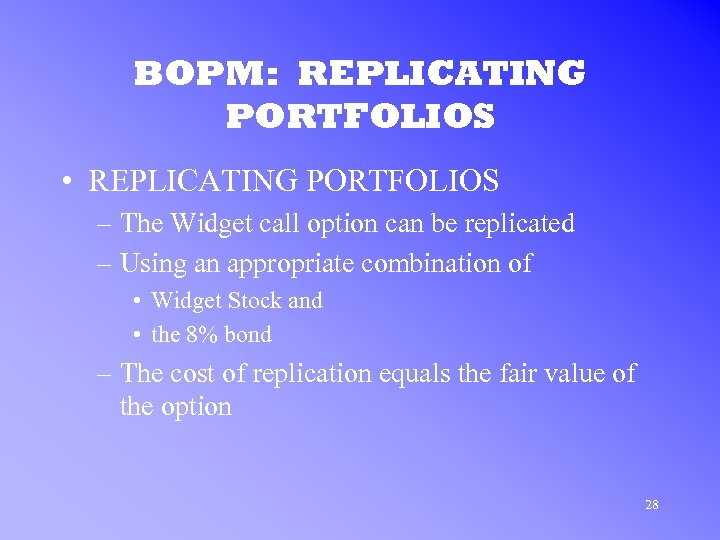BOPM: REPLICATING PORTFOLIOS • REPLICATING PORTFOLIOS – The Widget call option can be replicated – Using an appropriate combination of • Widget Stock and • the 8% bond – The cost of replication equals the fair value of the option 28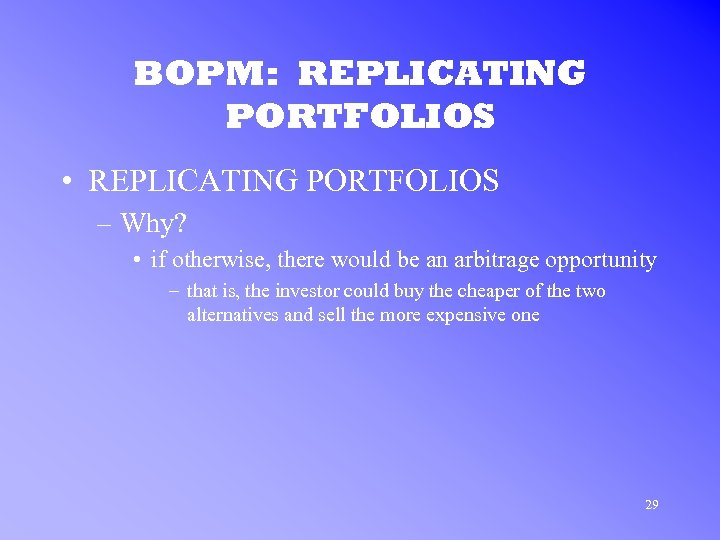BOPM: REPLICATING PORTFOLIOS • REPLICATING PORTFOLIOS – Why? • if otherwise, there would be an arbitrage opportunity – that is, the investor could buy the cheaper of the two alternatives and sell the more expensive one 29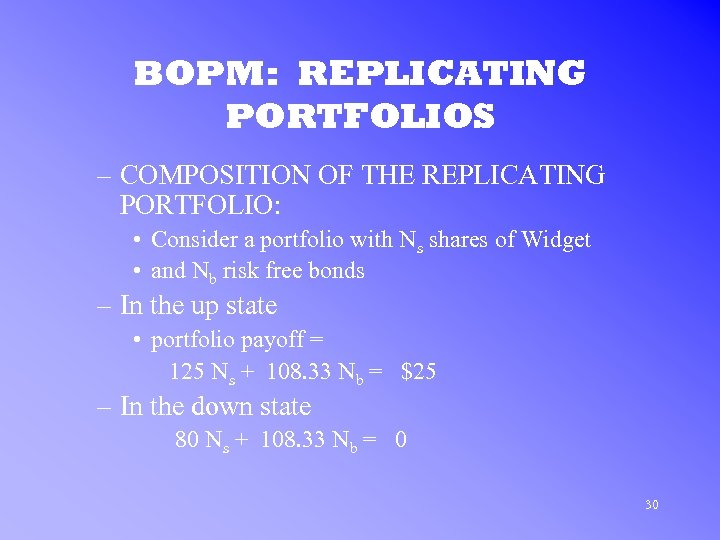BOPM: REPLICATING PORTFOLIOS – COMPOSITION OF THE REPLICATING PORTFOLIO: • Consider a portfolio with Ns shares of Widget • and Nb risk free bonds – In the up state • portfolio payoff = 125 Ns + 108. 33 Nb = \$25 – In the down state 80 Ns + 108. 33 Nb = 0 30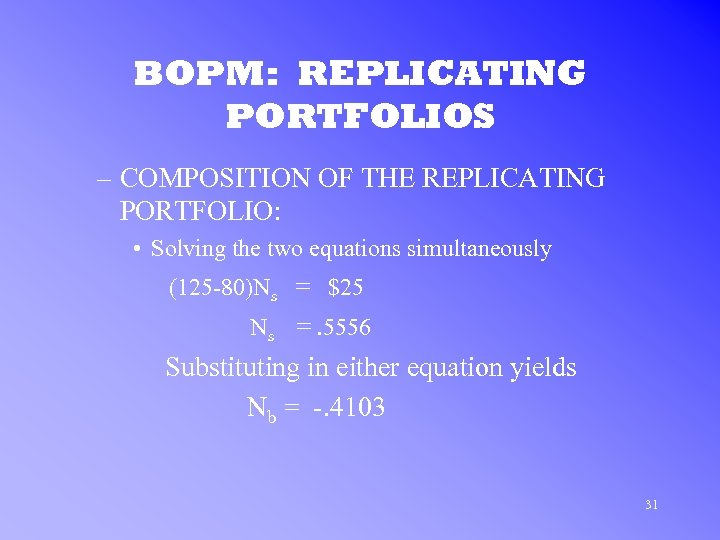BOPM: REPLICATING PORTFOLIOS – COMPOSITION OF THE REPLICATING PORTFOLIO: • Solving the two equations simultaneously (125 -80)Ns = \$25 Ns =. 5556 Substituting in either equation yields Nb = -. 4103 31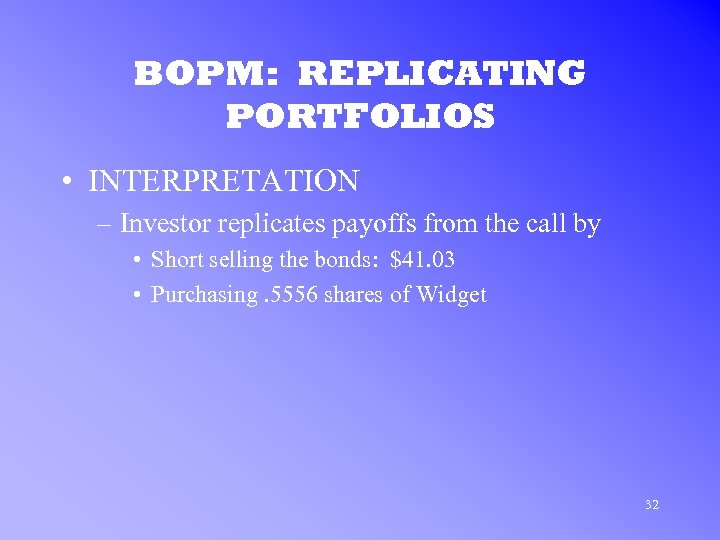BOPM: REPLICATING PORTFOLIOS • INTERPRETATION – Investor replicates payoffs from the call by • Short selling the bonds: \$41. 03 • Purchasing. 5556 shares of Widget 32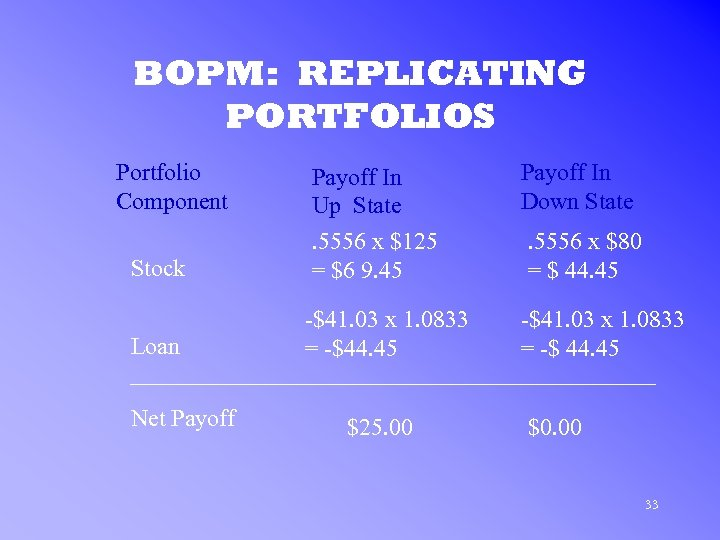BOPM: REPLICATING PORTFOLIOS Portfolio Component Stock Loan Net Payoff In Up State. 5556 x \$125 = \$6 9. 45 -\$41. 03 x 1. 0833 = -\$44. 45 \$25. 00 Payoff In Down State. 5556 x \$80 = \$ 44. 45 -\$41. 03 x 1. 0833 = -\$ 44. 45 \$0. 00 33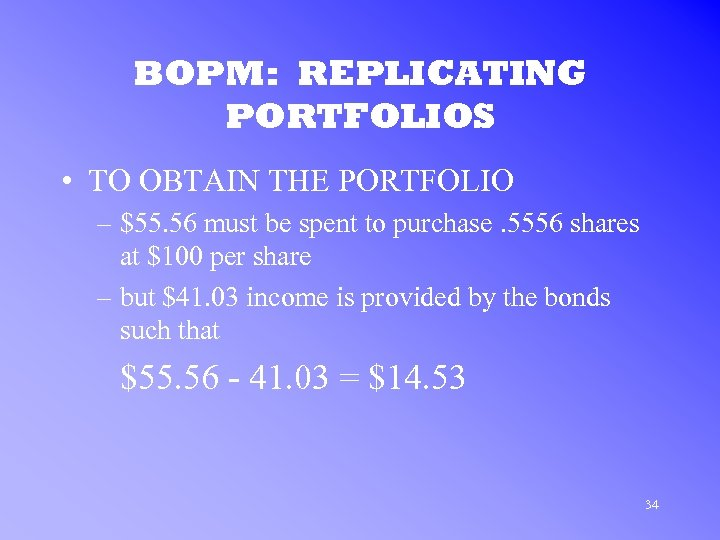BOPM: REPLICATING PORTFOLIOS • TO OBTAIN THE PORTFOLIO – \$55. 56 must be spent to purchase. 5556 shares at \$100 per share – but \$41. 03 income is provided by the bonds such that \$55. 56 - 41. 03 = \$14. 53 34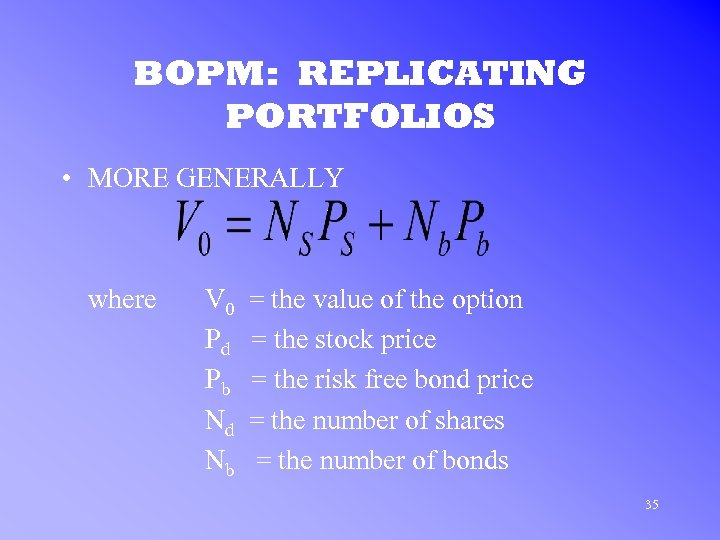BOPM: REPLICATING PORTFOLIOS • MORE GENERALLY where V 0 Pd Pb Nd Nb = the value of the option = the stock price = the risk free bond price = the number of shares = the number of bonds 35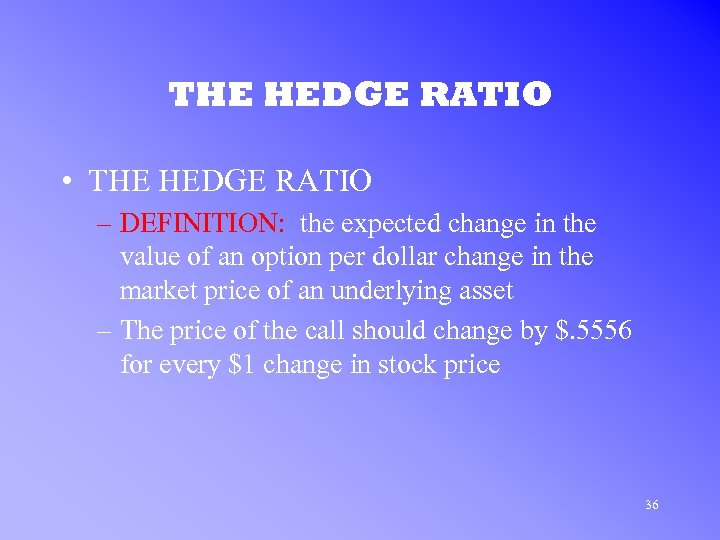THE HEDGE RATIO • THE HEDGE RATIO – DEFINITION: the expected change in the value of an option per dollar change in the market price of an underlying asset – The price of the call should change by \$. 5556 for every \$1 change in stock price 36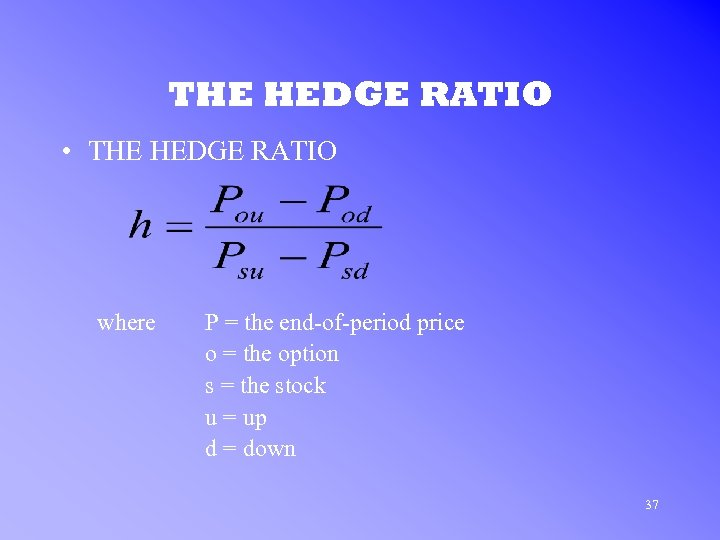THE HEDGE RATIO • THE HEDGE RATIO where P = the end-of-period price o = the option s = the stock u = up d = down 37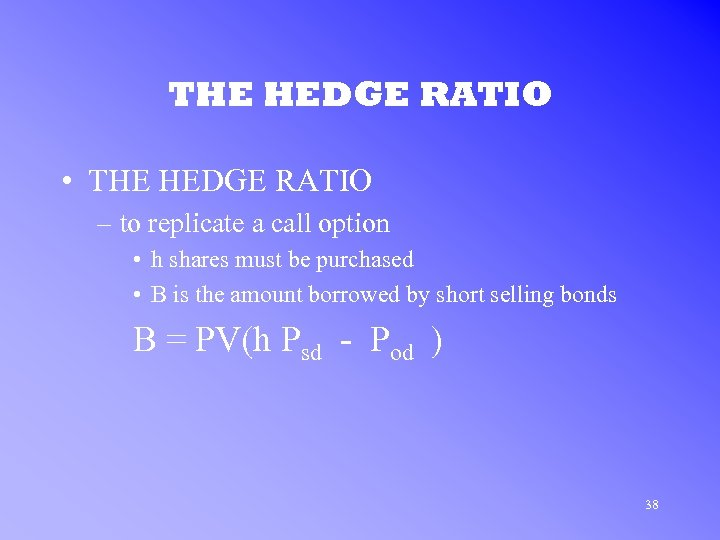THE HEDGE RATIO • THE HEDGE RATIO – to replicate a call option • h shares must be purchased • B is the amount borrowed by short selling bonds B = PV(h Psd - Pod ) 38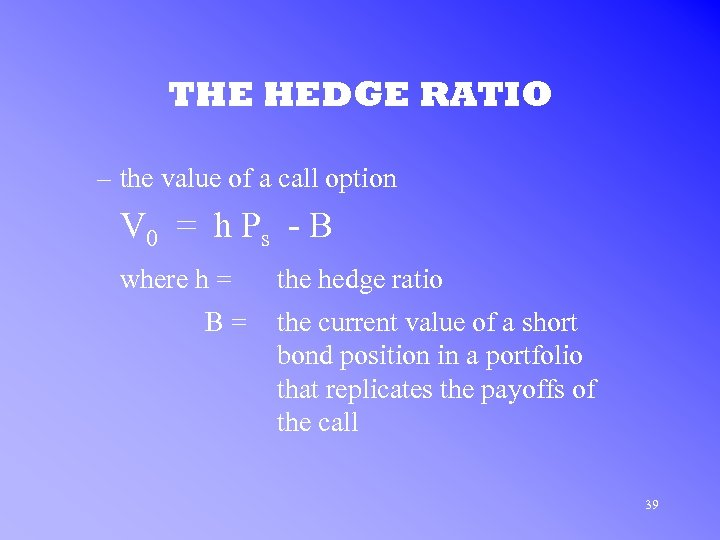THE HEDGE RATIO – the value of a call option V 0 = h P s - B where h = B= the hedge ratio the current value of a short bond position in a portfolio that replicates the payoffs of the call 39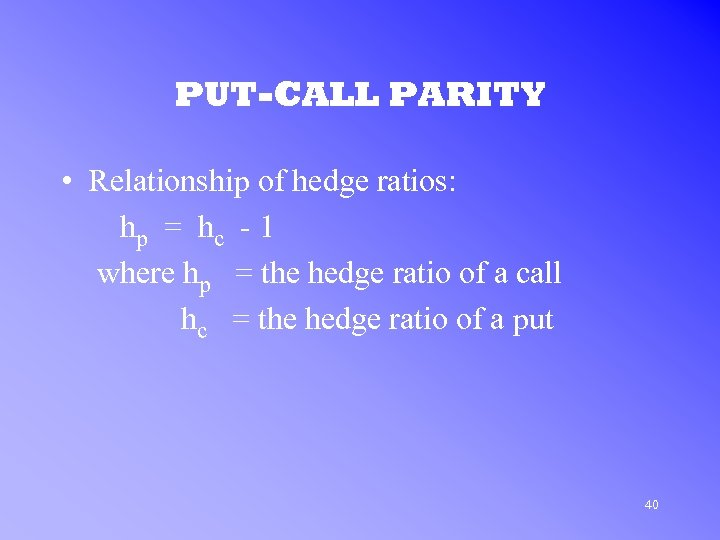PUT-CALL PARITY • Relationship of hedge ratios: hp = hc - 1 where hp = the hedge ratio of a call hc = the hedge ratio of a put 40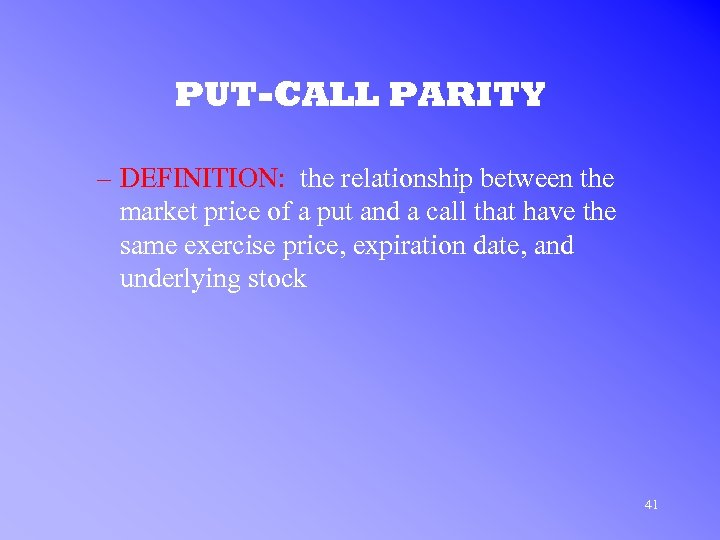PUT-CALL PARITY – DEFINITION: the relationship between the market price of a put and a call that have the same exercise price, expiration date, and underlying stock 41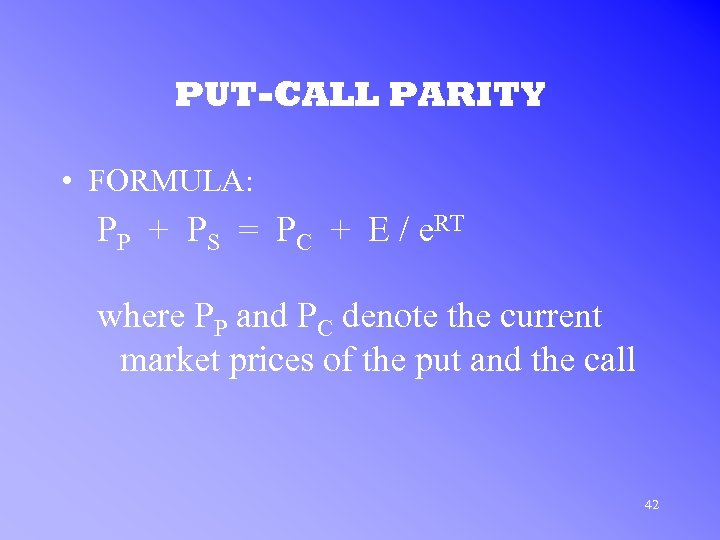PUT-CALL PARITY • FORMULA: PP + PS = PC + E / e. RT where PP and PC denote the current market prices of the put and the call 42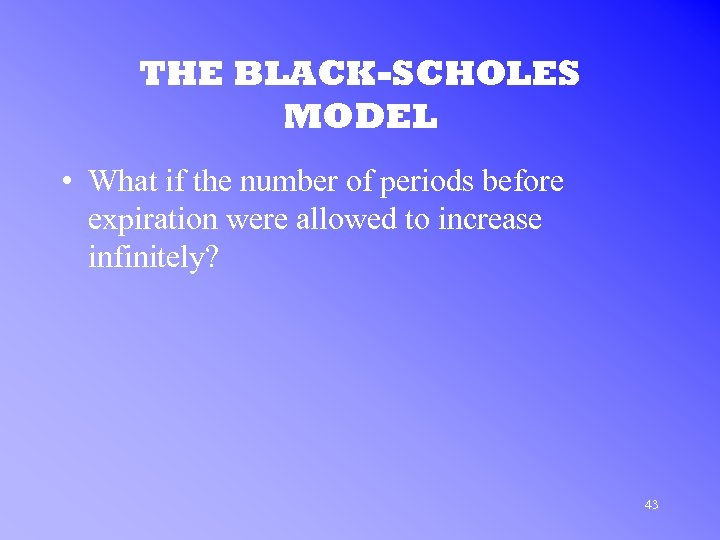THE BLACK-SCHOLES MODEL • What if the number of periods before expiration were allowed to increase infinitely? 43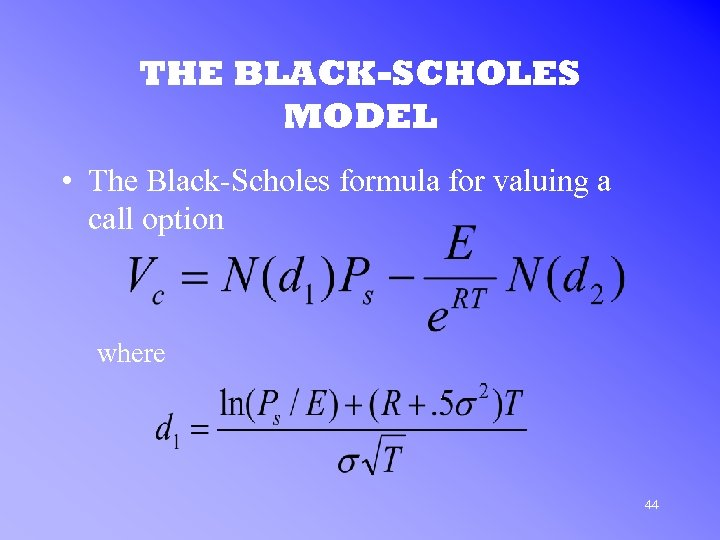THE BLACK-SCHOLES MODEL • The Black-Scholes formula for valuing a call option where 44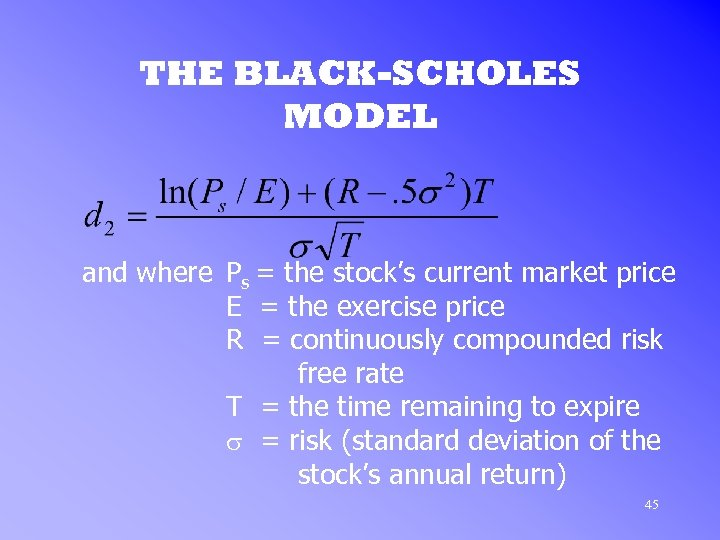THE BLACK-SCHOLES MODEL and where Ps = the stock’s current market price E = the exercise price R = continuously compounded risk free rate T = the time remaining to expire s = risk (standard deviation of the stock’s annual return) 45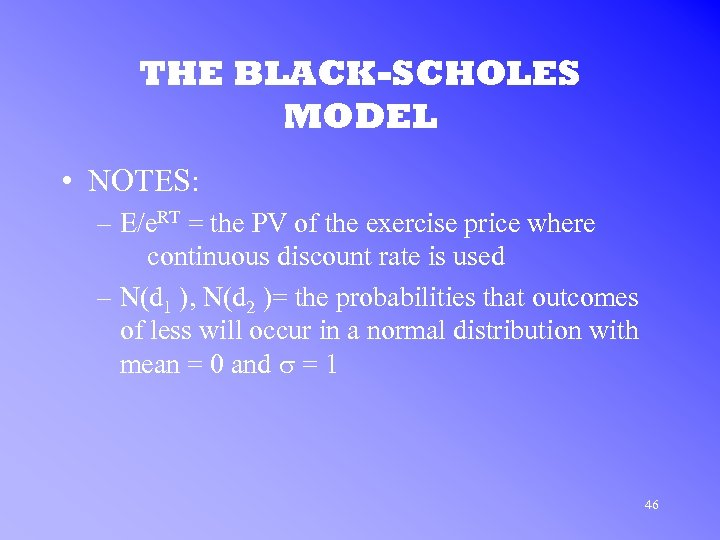THE BLACK-SCHOLES MODEL • NOTES: – E/e. RT = the PV of the exercise price where continuous discount rate is used – N(d 1 ), N(d 2 )= the probabilities that outcomes of less will occur in a normal distribution with mean = 0 and s = 1 46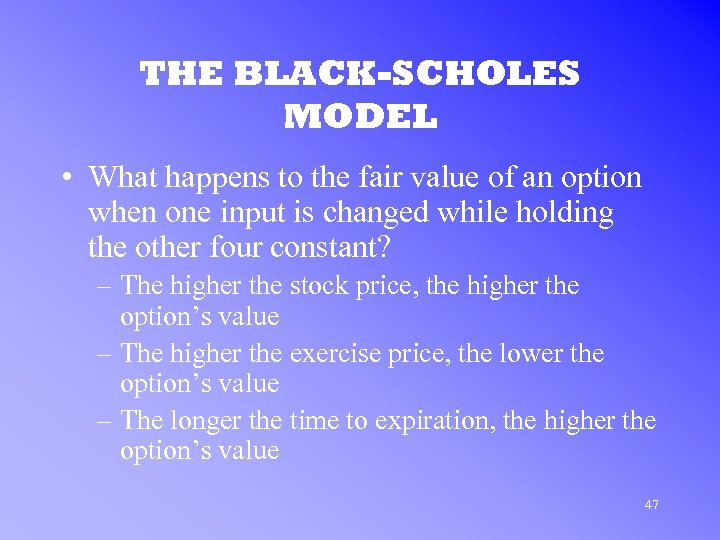THE BLACK-SCHOLES MODEL • What happens to the fair value of an option when one input is changed while holding the other four constant? – The higher the stock price, the higher the option’s value – The higher the exercise price, the lower the option’s value – The longer the time to expiration, the higher the option’s value 47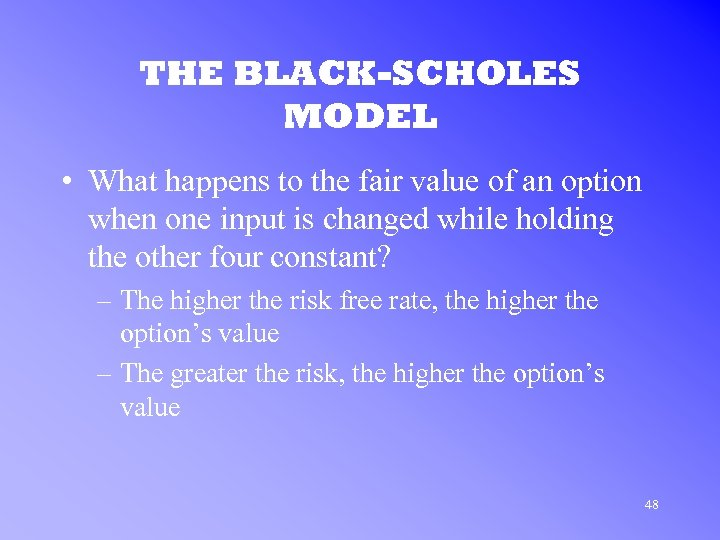THE BLACK-SCHOLES MODEL • What happens to the fair value of an option when one input is changed while holding the other four constant? – The higher the risk free rate, the higher the option’s value – The greater the risk, the higher the option’s value 48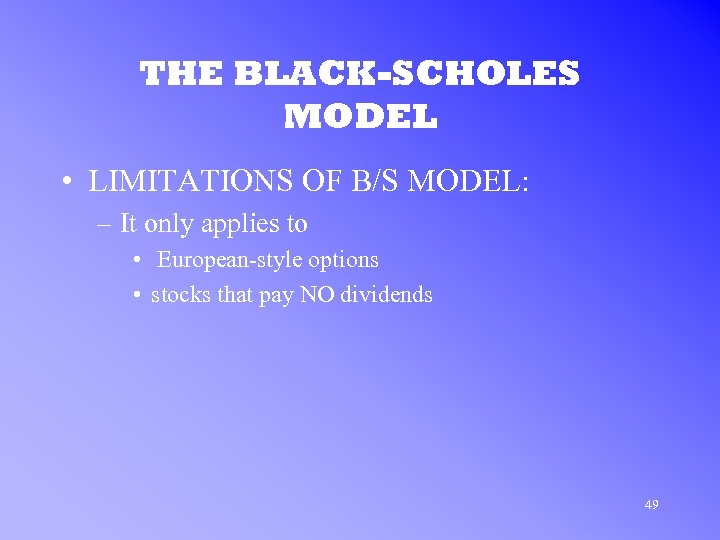THE BLACK-SCHOLES MODEL • LIMITATIONS OF B/S MODEL: – It only applies to • European-style options • stocks that pay NO dividends 49